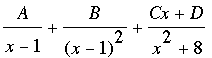SEARCH HOMEMath Central Quandaries & QueriesQuestion from taiwo, a student: i copied the question below from john bird's higher engineering mathematics fourth edition (pg 19) after solving all the partial fraction questions from the book except this: x^3 + 4^2 + 20x - 7 over (x-1)^2 (x^+8). i will appreciate it if you help me out so i can know whether their answer is correct. thanks alotHi Tiawo,

I think you mean x3 + 4x2 + 20 x - 7 over (x - 1)2(x2 + 8). The task is to write this in partial fraction form asWriting this sum of three fractions with a common denominator give a numerator which must equal the numerator of the original fraction. Thus

x3 + 4x2 + 20 x - 7 = A(x - 1)(x2 + 8) + B(x2 + 8) + (Cx + D)(x - 1)2

Substituting x = 1 in this equation gives B = 2 and hence the equation simplifies to

x3 + 2x2 + 20 x - 23
= A(x - 1)(x2 + 8) + (Cx + D)(x - 1)2
= (A + C)x3 + (A - 2C + D)x2 + (8a + C - 2D)x + (-8A + D)

Equating polynomial coefficients leads to the linear system

A  +  C        =    1
-A  - 2C +  D =   2
8A +  C - 2D =  20
-8A +         D = -23

This system yields A = 3, C = -2 and D = 1.

I hope this helps,
HarleyMath Central is supported by the University of Regina and The Pacific Institute for the Mathematical Sciences.